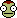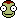# Orbital Mechanics Questions; Help

## Recommended Posts

Hey guys, understand this is a bit of an ask but I've come to the end of my tether with trying to calculate these.

If any of you are nerdy enough to give these a go I'd greatly appreciate it.

##### Share on other sites

Nice try, but we're not doing your homework.##### Share on other sites

I'll accept formulae, book suggestions and youtube tutorials people know of too.

##### Share on other sites

20 minutes ago, Daniel8520 said:

I'll accept formulae, book suggestions and youtube tutorials people know of too.

Simple. Google every question.

Google "L4 lagrange" to find out the relationship between earths orbital radius and the radius of the L4 point.

Google "orbital velocity calculator" and find an online calculator that will give you orbital velocities based on masses and altitudes.

Google any variable to find its relationship to known quantities.

Catch my drift?

Hint: playing KSP will help immensely in the understanding of all of these variablesEdited by p1t1o
##### Share on other sites

I can help a bit (I think, the little orbital mech I have is Keplerian rather than n-body):

1. Is the sun mass times G.
2. Not sure, haven't done LaGrange point radii before.
3. Will be the L4 orbit circumference divided by Earth's orbital period.
4. Is a unit conversion of a given in the problem (ax) from AU to m.
5. Can be calculated using the Vis Viva equation.
6. Is the sum of the science station radius and object radius divided by two.
7. Is twice the answer to 6.
8. Is calculated as negative gravitational parameter divided by twice the semi major axis of the transfer orbit.
9. Vis-viva again.
10. Answer to 9 minus the answer to 3.
11. I've forgotten angular momentum calcs, sorry.
12. Eccentricity has some calcs here, the e=f/a definition is most useful here (recall that the sun will be at one of the foci of the ellipse)
13. Vis-viva once more.
14. Not sure on this one.
##### Share on other sites

58 minutes ago, Red Iron Crown said:

I can help a bit (I think, the little orbital mech I have is Keplerian rather than n-body):

1. Is the sun mass times G.
2. Not sure, haven't done LaGrange point radii before.
3. Will be the L4 orbit circumference divided by Earth's orbital period.
4. Is a unit conversion of a given in the problem (ax) from AU to m.
5. Can be calculated using the Vis Viva equation.
6. Is the sum of the science station radius and object radius divided by two.
7. Is twice the answer to 6.
8. Is calculated as negative gravitational parameter divided by twice the semi major axis of the transfer orbit.
9. Vis-viva again.
10. Answer to 9 minus the answer to 3.
11. I've forgotten angular momentum calcs, sorry.
12. Eccentricity has some calcs here, the e=f/a definition is most useful here (recall that the sun will be at one of the foci of the ellipse)
13. Vis-viva once more.
14. Not sure on this one.

I thought #14 was a trick question with the answer being zero - both orbits are stated as co-planar, it is stated that the probe cannot be launched into a different elevation than the launching ship and no inclinations are given for any orbit or object...

##### Share on other sites

2 minutes ago, p1t1o said:

I thought #14 was a trick question with the answer being zero - both orbits are stated as co-planar, it is stated that the probe cannot be launched into a different elevation than the launching ship and no inclinations are given for any orbit or object...

The high energy transfer will be intercepting at a point other than its apoapsis, I took this angle to mean the difference between the probe's velocity vector and the object's.

##### Share on other sites

23 minutes ago, Red Iron Crown said:

The high energy transfer will be intercepting at a point other than its apoapsis, I took this angle to mean the difference between the probe's velocity vector and the object's.

I assumed that "elevation" references angle to the ecliptic, now Im not sure.

##### Share on other sites

There's actually a bit of flaw in the question regarding fast transfer orbit : generally speaking there'd only be a range of possible orbits and not a fixed one. So I guess it's asking about the minimum eccentricity orbit.

##### Share on other sites

This thread is quite old. Please consider starting a new thread rather than reviving this one.

## Join the conversation

You can post now and register later. If you have an account, sign in now to post with your account.
Note: Your post will require moderator approval before it will be visible.Reply to this topic...

×   Pasted as rich text.   Paste as plain text instead

Only 75 emoji are allowed.